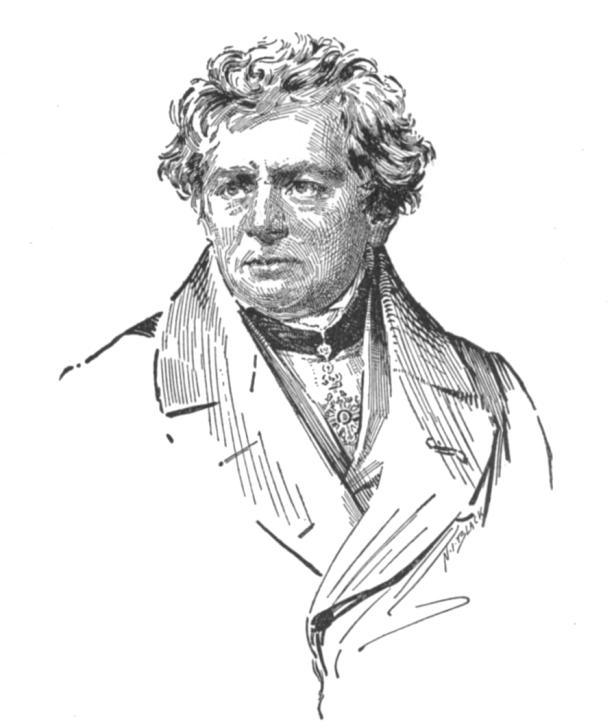# Science Site

## Ohm’s Law

Published on Jul 15, 2016

Tags:Keywords:, ,It is difficult to imagine a time when there was no electricity. In the modern world, it is hard to turn your head and not gaze upon something that utilized electricity. Here, electricity simply refers to the current that flows through an appliance or article, required to make the appliance work. Refrigerator, air conditioner, mobile charger, television – all function only when there is a current flowing through them.

On connecting these devices to the power socket there is a potential difference created which causes the flow of current. This potential difference can be understood in the same way as the potential difference between a full container of water and an empty container. If you connect these with the help of a pipe, water flows from the full container to the empty one when the full one is raised by some height.

### Statement of Ohm’s law

Ohm’s law states that the current which flows through a device or appliance is proportional to the potential difference or voltage that is applied. Remember that current is the rate of flow of electrons. The more the voltage, the more will be the current that flows. The same can be observed in the case of containers of water also. The more you raise the filled container of water, the faster the water flows into the empty container. Ohm’s law was given by Georg Ohm in 1827.

### Constant of proportionality

Ohm’s law defines a proportionality between current and voltage. In order to get a mathematical equation with an equal sign instead of a proportionality sign, there is a need for a constant of proportionality. In Ohm’s law, resistance offered by the circuit of a device to the current is defined as the constant of proportionality. Thus, the equation becomes:

V = I x R

When a certain amount of voltage is applied across the ends of a circuit, a current flows from one end to the other depending on the amount of resistance that is offered by the circuit. Resistance is an innate property of the circuit, it depends on the physical aspects of the circuit and cannot change. As the voltage is increased, the current also increases while resistance remains constant.

### Does Ohm’s law always hold good?

Ohm’s law does not always hold good. It is only valid for certain resistors which exhibit a linear dependence of voltage with current. A diode is an electrical device that does not show this kind of behaviour. When the voltage vs. current curve is plotted for a diode, the curve represents a non-linear relationship. These devices are called non-ohmic conductors while those that obey Ohm’s law are called ohmic conductor.

### Effect of temperature

Ohm’s law holds true at a constant temperature. When the temperature of a conductor is increased, the resistivity of the conductor changes. This change in resistivity or specific resistance causes a change in the resistance that the conductor offers to the current, and so Ohm’s law gets violated. Since the flow of current through any conductor leads to heating of the conductor, the Ohm’s law cannot be verified and stands violated when a large amount of current is made to flow through a device.